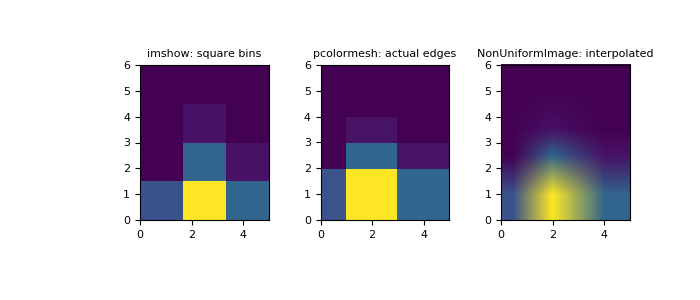# numpy.histogram2d¶

`numpy.``histogram2d`(x, y, bins=10, range=None, normed=False, weights=None)[source]

Compute the bi-dimensional histogram of two data samples.

Parameters: x : array_like, shape (N,) An array containing the x coordinates of the points to be histogrammed. y : array_like, shape (N,) An array containing the y coordinates of the points to be histogrammed. bins : int or array_like or [int, int] or [array, array], optional The bin specification: If int, the number of bins for the two dimensions (nx=ny=bins). If array_like, the bin edges for the two dimensions (x_edges=y_edges=bins). If [int, int], the number of bins in each dimension (nx, ny = bins). If [array, array], the bin edges in each dimension (x_edges, y_edges = bins). A combination [int, array] or [array, int], where int is the number of bins and array is the bin edges. range : array_like, shape(2,2), optional The leftmost and rightmost edges of the bins along each dimension (if not specified explicitly in the bins parameters): `[[xmin, xmax], [ymin, ymax]]`. All values outside of this range will be considered outliers and not tallied in the histogram. normed : bool, optional If False, returns the number of samples in each bin. If True, returns the bin density `bin_count / sample_count / bin_area`. weights : array_like, shape(N,), optional An array of values `w_i` weighing each sample `(x_i, y_i)`. Weights are normalized to 1 if normed is True. If normed is False, the values of the returned histogram are equal to the sum of the weights belonging to the samples falling into each bin. H : ndarray, shape(nx, ny) The bi-dimensional histogram of samples x and y. Values in x are histogrammed along the first dimension and values in y are histogrammed along the second dimension. xedges : ndarray, shape(nx+1,) The bin edges along the first dimension. yedges : ndarray, shape(ny+1,) The bin edges along the second dimension.

`histogram`
1D histogram
`histogramdd`
Multidimensional histogram

Notes

When normed is True, then the returned histogram is the sample density, defined such that the sum over bins of the product `bin_value * bin_area` is 1.

Please note that the histogram does not follow the Cartesian convention where x values are on the abscissa and y values on the ordinate axis. Rather, x is histogrammed along the first dimension of the array (vertical), and y along the second dimension of the array (horizontal). This ensures compatibility with `histogramdd`.

Examples

```>>> import matplotlib as mpl
>>> import matplotlib.pyplot as plt
```

Construct a 2-D histogram with variable bin width. First define the bin edges:

```>>> xedges = [0, 1, 3, 5]
>>> yedges = [0, 2, 3, 4, 6]
```

Next we create a histogram H with random bin content:

```>>> x = np.random.normal(2, 1, 100)
>>> y = np.random.normal(1, 1, 100)
>>> H, xedges, yedges = np.histogram2d(x, y, bins=(xedges, yedges))
>>> H = H.T  # Let each row list bins with common y range.
```

`imshow` can only display square bins:

```>>> fig = plt.figure(figsize=(7, 3))
>>> ax = fig.add_subplot(131, title='imshow: square bins')
>>> plt.imshow(H, interpolation='nearest', origin='low',
...         extent=[xedges, xedges[-1], yedges, yedges[-1]])
```

`pcolormesh` can display actual edges:

```>>> ax = fig.add_subplot(132, title='pcolormesh: actual edges',
...         aspect='equal')
>>> X, Y = np.meshgrid(xedges, yedges)
>>> ax.pcolormesh(X, Y, H)
```

`NonUniformImage` can be used to display actual bin edges with interpolation:

```>>> ax = fig.add_subplot(133, title='NonUniformImage: interpolated',
...         aspect='equal', xlim=xedges[[0, -1]], ylim=yedges[[0, -1]])
>>> im = mpl.image.NonUniformImage(ax, interpolation='bilinear')
>>> xcenters = (xedges[:-1] + xedges[1:]) / 2
>>> ycenters = (yedges[:-1] + yedges[1:]) / 2
>>> im.set_data(xcenters, ycenters, H)
>>> ax.images.append(im)
>>> plt.show()
```numpy.histogram

#### Next topic

numpy.histogramdd